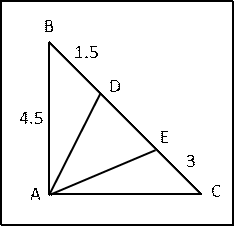# Math Book 3: Geometry

You may get two to three questions from Geometry in the GMAT quant section. Usually tested as a problem solving variant, you could occassionally come across a data sufficiency question testing core concepts in geometry.

### Concepts Covered

Basics of geometry including properties of triangles, similar triangles, quadrilaterals, polygons and circles are often tested in the GMAT Math Section. Make sure that you do not just memorize the formulae or the theorem. Though you will never be tested on derivations of the theorem, going through the derivations and proofs helps understand concepts better and therefore, solve questions with ease. Wizako's GMAT Math Lesson Book in this chapter covers the following concepts:

1. Definition of various terms used in geometry
2. Properties and theorems of lines, angles and parallel lines
3. Basic proportionality theorem
4. Properties of triangles explained with illustrative examples
5. Different types of triangles
6. Concepts and theorems, such as Apollonius Theorem and Pythagoras Theorem, associated with triangles with illustrative examples
8. Definition and properties of median, altitude, perpendicular bisector and angle bisector of a triangle
9. Introduction to different polygons including quadrilaterals such as squares, rectangles, rhombus, parallelogram, kite and trapezium.
10. Circles and properties of circles with illustrative examples
11. Chords, tangents, secants, circles touching externally, circles touching internally, intersecting circles.
12. 10 illustrative examples and 33 solved examples.
14. A multiple choice question test with 52 GMAT level questions in the work book. An answer key and explanatory answer for all questions are provided.

Here is a typical solved example from this chapter.

### Sample Question

In $$triangle$ABC. AB = 4.5, AC = 6 and BC = 7.5. The points D & E lie on the line segment BC with BD = 1.5 and EC = 3. What is the value of the ∠DAE? #### Explanatory AnswerThe triangle is right-angled$4.5, 6 and 7.5 is a 3k, 4k, 5k Pythagoras triplet where k = 1.5).
Therefore, ∠A = 900.
BC = 7.5, BD = 1.5, and EC = 3. Therefore, BE = 4.5 and DC = 6
Hence, AB = BE = 4.5 and AC = CD = 6 and

$$triangle$ABE is an isosceles triangle.$Two sides AB and BE are equal)
Therefore, corresponding angles opposite to AB and BE will be equal. i.e., ∠BAE = ∠BEA = ∠DEA (∠BEA is the same as ∠DEA )

Similarly, $$triangle$ ACD is also an isosceles triangle.$Two sides AC and DC are equal).
So, corresponding angles opposite to AC and DC will be equal. i.e., ∠DAC = ∠ADE

=> ∠DAC + ∠BAE + ∠DAE = 180o
=> (90o + ∠DAE) + ∠DAE = 180o
=> ∠DAE = 45o

### Chapterwise details of Wizako's Math Lesson Books

Math Lesson Book 1

 1 Linear Equations Details 2 Quadratic Equations Details 3 Set Theory Details 4 Sequences & Series Details 5 Number Properties & Theory Details 6 Inequalities Details 7 Functions

Math Lesson Book 2

 1 Descriptive Statistics Details 2 Ratio Proportion Details 3 Mixtures 4 Interest 5 Rates: Speed Distance Details 6 Rates: Races Details 7 Rates: Work Time Details

Math Lesson Book 3

 1 Percents Details 2 Profits Details 3 Permutation Combination Details 4 Probability Details 5 Geometry Details 6 Solid Geometry 7 Coordinate Geometry Details